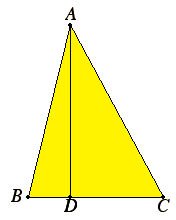# Proposition 13

In acute-angled triangles the square on the side opposite the acute angle is less than the sum of the squares on the sides containing the acute angle by twice the rectangle contained by one of the sides about the acute angle, namely that on which the perpendicular falls, and the straight line cut off within by the perpendicular towards the acute angle.
I.12

Let ABC be a triangle having the angle at B acute, and draw AD from the point A perpendicular to BC.

I say that the square on AC is less than the sum of the squares on CB and BA by twice the rectangle CB by BD.II.7

Since the straight line CB has been cut at random at D, the sum of the squares on CB and BD equals twice the rectangle CB by BD plus the square on DC.

Add the square on DA to each. Therefore the sum of the squares on CB, BD, and DA equals twice the rectangle CB by BD plus the sum of the squares on AD and DC.

I.47

But the square on AB equals the sum of the squares on BD and DA, for the angle at D is right, and the square on AC equals the sum of the squares on AD and DC, therefore the sum of the squares on CB and BA equals the square on AC plus twice the rectangle CB by BD, so that the square on AC alone is less than the sum of the squares on CB and BA by twice the rectangle CB by BD.

Therefore in acute-angled triangles the square on the side opposite the acute angle is less than the sum of the squares on the sides containing the acute angle by twice the rectangle contained by one of the sides about the acute angle, namely that on which the perpendicular falls, and the straight line cut off within by the perpendicular towards the acute angle.

Q.E.D.

## Guide

See the guide for the previous proposition II.12.